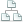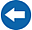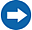Home >Tips >Excel 2016 from Scratch >Calculate Maximum, Minimum and AverageSite map

# Excel 2016 from Scratch Exercise Calculate Maximum, Minimum and Average

## An Excel tutorial by Peter KalmstromIn this demo in the Excel 2016 from Scratch series, Peter Kalmstrom shows two different ways to calculate the maximum, minimum and average values from cells with numbers. As an example Peter uses the price and mileage of cars of different brands and from different years.

Peter shows two methods, one where he selects the cell range for each formula and one where he instead gives a name to the cell range and uses it in the formula.

### Exercise

If you want to try the exercise yourself, please download the Excel file Peter uses in the demo!

### Content

This is what Peter shows in the demo below:

• How to find the minimum value among several cells with values by using a formula and select the cells that should be included.
• How to find the maximum value among several cells with values by using a formula and select the cells that should be included.
• How to calculate the average value of several cell values by using a formula and select the cells that should be included.
• How to give a name to a selected range of cells.
• How to find the minimum value among several cells with values by using a formula where the range name is included.
• How to find the maximum value among several cells with values by using a formula where the range name is included.
• How to calculate the average value of several cell values by using a formula where the range name is included.
• How to display numbers in different styles.
Peter uses Excel 2016 for his demo, but the Excel basics are the same for earlier versions of Excel.